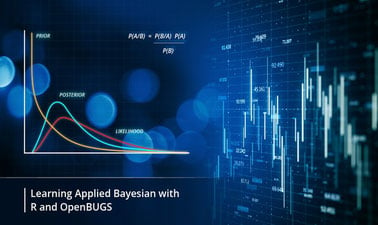# Applied Bayesian for Analytics

Learn how to construct, fit, estimate and compute Bayesian statistical models with the help of OpenBUGS (freely available software)This course is archived
Estimated 6 weeks
2–3 hours per week
Instructor-paced
Instructor-led on a course schedule
Free

Bayesian Statistics is a captivating field and is used most prominently in data sciences. In this course we will learn about the foundation of Bayesian concepts, how it differs from Classical Statistics including among others Parametrizations, Priors, Likelihood, Monte Carlo methods and computing Bayesian models with the exploration of Multilevel modelling.

This course is divided into two parts i.e. Theoretical and Empirical part of Bayesian Analytics. First three weeks cover the Theoretical part which includes how to form a prior, how to calculate a posterior and several other aspects. Rest of the weeks will cover the empirical part which explains how to compute Bayesian modelling. Completion of this course will provide you with an understanding of the Bayesian approach, the primary difference between Bayesian and Frequentist approaches and experience in data analyses.

### At a glance

• Language: English

# What you'll learn

Skip What you'll learn
• Understand the necessary Bayesian concepts from practical point of view for better decision making.
• Learn Bayesian approach to estimate likely event outcomes, or probabilities using datasets.
• Gain “hands on” experience in creating and estimating Bayesian models using R and OPENBUGS.

# Syllabus

Skip Syllabus

Week 01: What is Bayesian Statistics and How it is different than Classical Statistics

• Foundations of Bayesian Inference
• Bayes theorem
• Why Bayesian approach is so important in Analytics
• Major densities and their applications

Week 02: Bayesian analysis of Simple Models

• Likelihood theory and Estimation
• Parametrizations and priors
• Learning from binary models
• Learning from Normal Distribution

Week 03: Monte Carlo Methods

• Basics of Monte carol integration
• Basics of Markov chain Monte Carlo
• Gibs Sampling

Week 04: Computational Bayes

• Examples of Bayesian Analytics
• Introduction to R and OPENBUGS for Bayesian analysis

Week 05: Bayesian Linear Models

• Context for Bayesian Regression Models
• Normal Linear regression
• Logistic regression

Week 06: Bayesian Hierarchical Models

• Introduction to Multilevel models
• Exchangeability
• Computation in Hierarchical Models